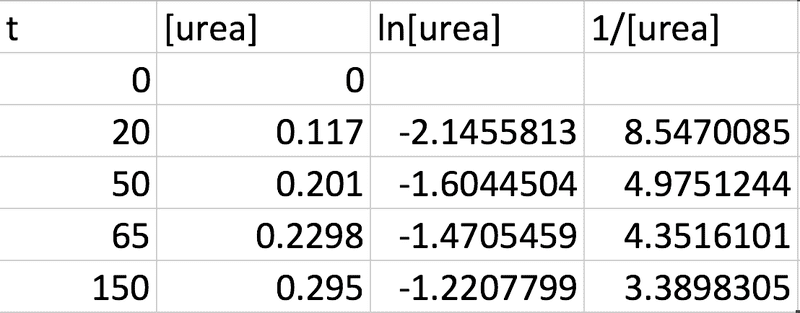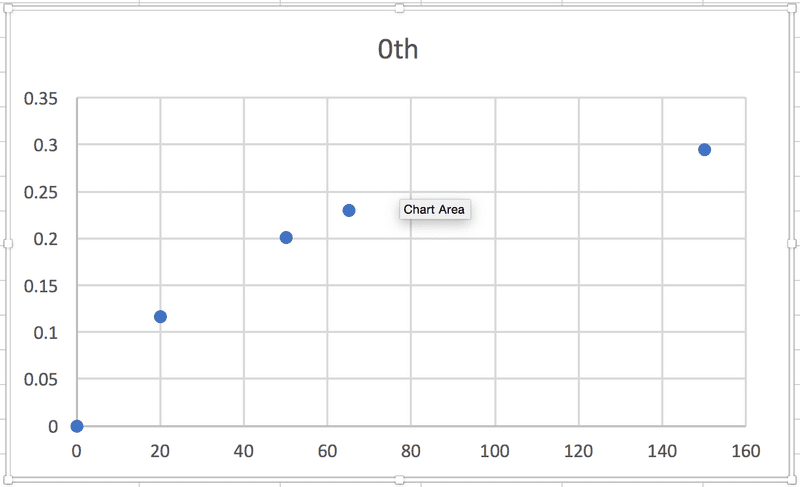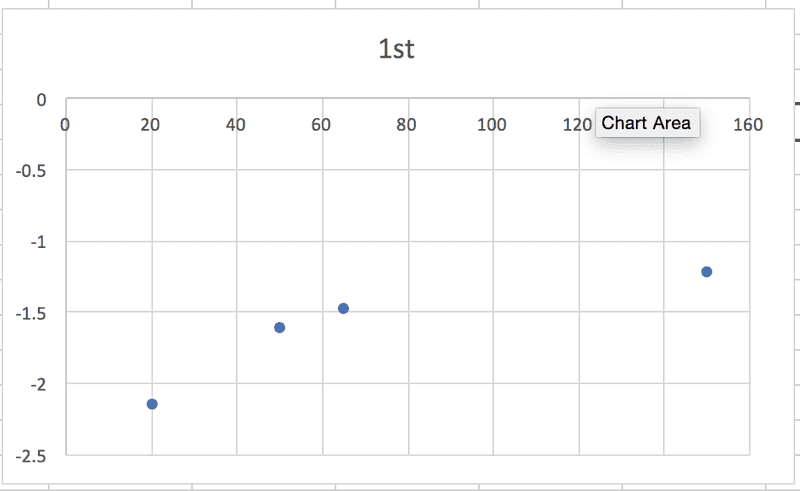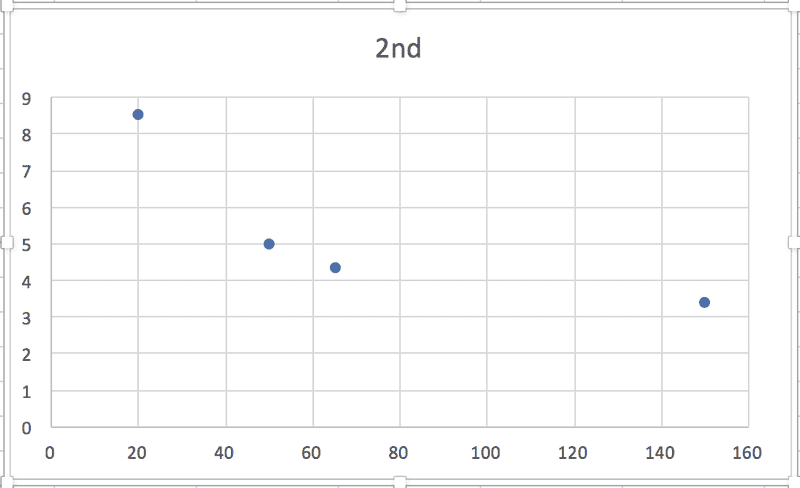# Finding order of reaction & rate constant

Ryaners
I'm trying to solve one of the problems in my textbook (Atkins Physical Chemistry) and I just can't get it out. Here's the problem:

"The data below apply to the formation of urea from ammonium cyanate. Initially 22.9g of ammonium cyanate was dissolved in enough water to prepare 1.00 dm3 of solution. Determine the order of the reaction , the rate constant, and the mass of ammonium cyanate left after 300min.

t / min: 0 20.0 50.0 65.0 150
mass / g: 0 7.0 12.1 13.8 17.7 "

From the question I know that the concentration will be equal in magnitude to the mass / amount in moles as the volume is 1 dm3. So based on this assumption I calculated the following from the given data (taking the molar mass of urea to be 60.06g):This is based on the idea that the integrated rate laws for unimolecular reactions are:

0th: [urea]t = [urea]0 - kt

1st: ln[urea]t = ln[urea]0 -kt

2nd: 1 / [urea]t = 1 / [urea]0 + kt

So, in each case, the relationship between some particular expression of [urea] and t is linear, which makes sense to me, and the slope of the graph will be equal to -k. (Or +k in this case, I think, because it's the product concentration I'm given.) BUT when I graph each of the sets of data against time, none of them are linear:According to the textbook, the reaction is 2nd order. Where am I going wrong?! Thanks in advance for any help.

Homework Helper
Gold Member
Not sure but I think you might have seen the problem (or it would have been clearer to us) if you had labelled your ordinates. What's are they? - urea amounts/concentrations?

You appear to be doing stuff in function of urea concentrations, but the rate most likely depends only on ammonium cyanate concentration which you can obtain from the urea concentration and plot functions of.

•Ryaners
Ryaners
Not sure but I think you might have seen the problem (or it would have been clearer to us) if you had labelled your ordinates. What's are they? - urea amounts/concentrations?

You appear to be doing stuff in function of urea concentrations, but the rate most likely depends only on ammonium cyanate concentration which you can obtain from the urea concentration and plot functions of.

Yes! That's it, thank you so much! You wouldn't believe how long I spent tearing my hair out over this yesterday. Learning is hard.I'm a bit confused about something I think. I've learned that the rate of formation of product = - rate of consumption of reactant (which makes sense), but also that the rate can be affected by the concentration of reactant in a different way than by the formation of product, like in this problem. I'm having a hard time getting my head around how you can produce something at the same rate as another thing is consumed - the rate is the same (but opposite in sign) - but that rate is not affected by the change in concentration in one thing, while is IS affected by the change in concentration in the other. Any advice on understanding that better?Homework Helper
Gold Member
Well for study and problem-solving I think tension and worry are negative so I hope you ca get a way of relaxing, and standing back from a problem or subject and thinking "what is this really about?" Or "what is the book trying to say about this subject"?.

For your question In the simplest case of an irreversible reaction A → B well the A molecules just split , rearrange or whatever and form B and that's it, each A molecule having an equal chance of doing that then the rate is expected to be proportional to [A]. In simplest mechanisms pretty well summarized by A → B no reason [ B] should influence the rate even though the concentrations are related by [A] + [ B] = [A]0, a constant quantity. If instead the reaction is reversible it goes both ways and necessarily the product also influences reaction rate. However it is going both ways at the same time in the passage towards equilibrium, so you only see one reaction in one direction. Only one change. You can measure either change of [A] or of [ B] whichever is more convenient, both is superfluous. You will soon find the kinetics is formulated in terms of only one concentration, usually whatever is present at the start. The above applies really only to simple reaction mechanisms but will do for now.

If I had to guess I would guess 2nd order kinetics for this reaction because I guess it is a reaction between two ions NH4+ and CNO- ...

Since you have to plot and calculate a rate constant anyway it would be good if you could show your results and conclusions here too so we can mark a problem as solved for once.

Last edited: